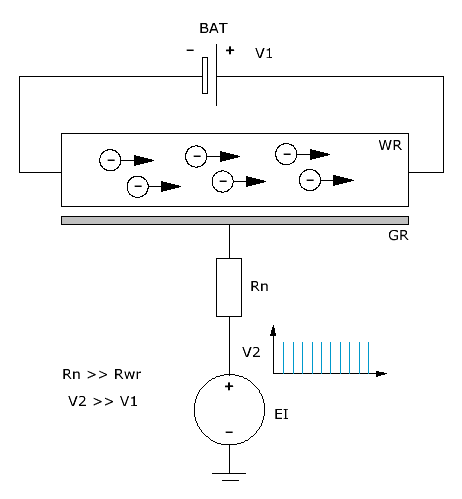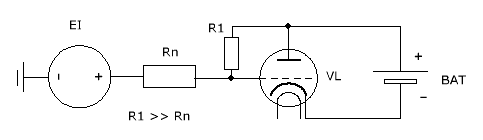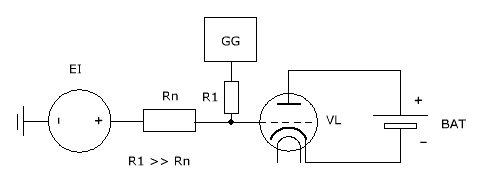Research website of Vyacheslav Gorchilin
2016-06-21
Lick-a method of improving the efficiency of the second kind
Method is available for joint patenting

At the junction of two branches of physics — electrostatics and electrodynamics — one can observe a very interesting and unusual from their point of view, the effects and phenomena. Next, we will discuss one of them, it will build a simple mathematical model and propose some circuit solutions.

The article about free energy was considered some techniques to improve the efficiency of the devices and the efficiency of the second kind\eta_{2}. Here we present one such method. It is characterized by its simplicity and relatively simple circuitry for its implementation. Why this method is so named, it will be clear from further discussion :)

For understanding of this we will consider the ideal case, which does not take into account the various losses (heat, radiation), which implies a maximum energy output at matched load R_{n}. In the beginning, consider a channel with a current WR. In fact, this kind of environment with its internal resistance R_{WR}, in which the moving negative charges from the cathode (left) to the anode (right). The direction and magnitude of the current defines the power source BAT. For our ideal case we consider that its internal resistance is zero.

The idea of a lick-the method lies in the switching movement of a portion of the charges from the circuit BAT - WR - BAT on a chain WR - R_{n} - EI due to the higher pulse voltage on a removable grid GR. Again, ideally, the duration between such switches must exactly match the time of filling of the channel charges.Calculate the efficiency of this setup for the perfect event. For this, we consider two chains: BAT - WR and WR - R_{n} - EI, as well as two kinds of variables: impulse and average for the period, for example V_{2imp} and V_{2avg}. Assume that when applying the HV pulse from the source EI portion of the charge completely flows through R_{n}. To do this, the duty cycle of the pulse Q_{EI} must be proportional to the average voltage value of this source:
Q_{EI} = \frac {V_{2avg}} {V_{1avg}}
Since the amount of charge in the period in both circuits are the same, and the average value of current in these circuits will also be identical:
I_{2avg} = I_{1avg}
The active power in the first circuit is as:
P_{1avg} = I_{1avg} V_{1avg}
The active power in the second circuit:
P_{2avg} = I_{2avg} V_{2avg}
Since I_{1avg} and I_{2avg} are equal, we find that the effectiveness of the device or increase the efficiency of the second kind is as follows:
K_{\eta2} = \frac {P_{2avg}} {P_{1avg}} = Q_{EI}
It would seem that endlessly increasing the duty cycle, we can proportionally increase the effectiveness of the device, but in this ideal case, we find that the pulse voltage should increase at the same time in a quadratic dependence:
V_{2imp} = V_{2avg} Q_{EI} = (V_{1avg} Q_{EI}) Q_{EI} = V_{1avg} Q_{EI}^{2}
For example, if the duty cycle is 10, and the voltage on the BAT — 100 Volts, the pulse voltage EI must be equal to 10'000 Volts. This voltage can not stand all the devices, and this point must be taken into account when designing the actual device.
For completeness, we find the ratio of the resistances of the load R_{n} and channel R_{WR}, which is found from the previous formulae:
\frac {R_{n}} {R_{WR}} = Q_{EI}
As you can see, the duty cycle Q_{EI} determines all the parameters of the scheme and to obtain the desired effect must be much greater than unity.
Real device

After all of the above immediately suggests a simple scheme of the real device. In it, as a channel, and a removable grid used tube with its anode, cathode and grid. The displacement on the grid that specifies the amount of channel resistance is set with R1. From the source EI receives high voltage pulses which, together with a load of Rn and give an increase of power:The disadvantage of the previous scheme — a fairly high frequency generator EI. If he's got the repetition period of pulses will be greater than required to fill the channel charges, there will be losses in the form of the current through the cathode-anode lamp. From here, you can leave, if you add another generator GG, which will work simultaneously with EI, but will supply the bias voltage just before the appearance of the HV pulse:It is worth noting that at the lick method, you can develop the circuitry and transistors and p-n-p transition can replace the lamp. The advantage of this approach may be under voltage BAT is of the order of Volts, and a sufficiently large pulse on EI — hundreds of volts, which will allow to achieve relatively large values of duty cycle and, thus, the efficiency of the entire device.

If in the future will be developed conductive materials with a super low output energy of the electron, described here the device will have millimeter dimensions.

The materials used